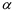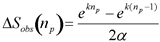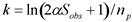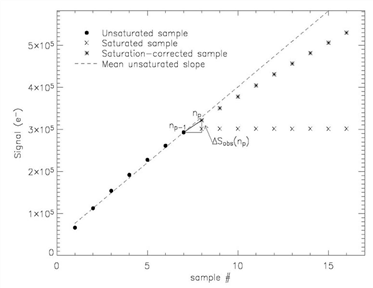Spitzer Documentation & Tools
IRS Instrument Handbook

### 5.1.5             SATCOR

SATCOR (�Saturation Correction�) operates on the data by replacing saturated samples with extrapolations from the non-saturated samples in the ramp. By �saturation� we refer to A/D (analog-to-digital) saturation, as identified by the CVTI2R4 module. The algorithm consists of fitting a slope to the non-saturated samples of a ramp and then using this slope to predict the values of saturated samples (see Figure 5.4). The outputs of this module (satcor.fits and satcor_unc.fits) are used only in the subsequent two modules, DROOPOP and ROWDROOP, to estimate the total charge on the array, using the saturation-corrected samples. Otherwise, the output of SATCOR is not propagated further downstream in the pipeline.

The first step in SATCOR consists of calculating the ramp slope of each pixel. This slope is the mean of slopes between pairs of consecutive samples, after excluding radhits.

In general (see below for some exceptions) SATCOR uses the last unsaturated sample and the calculated mean slope of the ramp up to that point to extrapolate the rest of the saturated ramp.

SATCOR requires as input the non-linearity coefficientfor each pixel, which is read from the lincal.fits calibration file. In practice, the sameis used for all pixels in a given module. The exception is the SL module, where the peak-up arrays may have a different coefficient than the rest of the array. The coefficientsare used by SATCOR to impart non-linearity (curvature) to the portions of the ramps that are saturation-corrected by the module. The first equation in the LINEARIZ module description (Section 5.1.11) expresses the observed (non-linear) signal in the ramp, Sobs, as an exponential function of ideally linear signal Slin. The latter is a linear function of plane number np, so that  the change in observed signal Sobs from plane np − 1 to np is given by the following equation, provided there were no radhits or saturation in planes np or np-1 for the given pixel.Equation 5.4

The constantEquation 5.5

can be evaluated at the first plane (np= 1), by assigning to Sobs the value of the mean slope of the ramp.

In some special circumstances, SATCOR does not replace the saturated value with the computed linearized extrapolation. These are:

1) If the pixel is fatally flagged in the pmask, or if all samples of the ramp are saturated, or if the first non-skipped sample is saturated, then all samples of the SATCOR output ramp are set to NaN.

2) Samples which have previously been identified and flagged as radhits within a ramp will be passed to DROOPOP unchanged.

The output file of this module is satcor.fits. This file is not available in the Spitzer Heritage Archive, but it can be generated by running CUPID. For every sample that is saturation-corrected in satcor.fits, the dmask.fits corresponding sample is updated by setting bit # 4.Figure 5.4 This ramp exhibits A/D saturation in samples 8-16 (x). SATCOR outputs saturation-corrected samples (asterisks). The operation consists of first obtaining the mean slope of the unsaturated portion of the ramp (dashed line). This slope is then used to extrapolate the signal from the last unsaturated sample (indexed as np-1), to the saturated sample immediately following (np). The calculation takes into account non-linearity of a realistic ramp, so that the extrapolated sample np will be smaller than if it were along the dashed line. The extrapolation is done in succession for higher samples up to the top of the ramp. The saturation-corrected samples are used only by the subsequent modules DROOP and ROWDROOP.